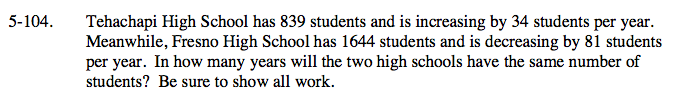### Home > GC > Chapter 5 > Lesson 5.3.4 > Problem5-104

5-104.Define the variables. Write the equations for the populations of the schools over time in y = mx + b form.

The equation that represents the population of Tehachapi High School over time is y = 34x + 839, because the growth is 34 students (m) and it started at 834 students (b). Now write the equation for Fresno High.

Once you have both equations you have a system of equations. So solve by using the Equal Values method.